# Evaluation

I began writing this chapter because I got a mail suggesting that I explain why `square-root 16 + square-root 16` is not 8. At first I figured out the "3 concepts" explanation below. This was supposed to be a simple chapter, almost a footnote. It turned out to be the chapter I spent most time writing and rewriting, as discussions in gitter pointed at different simple rules to describe the evaluation process. I'm not sure I agree with some of them.

I have a feeling I'll have to rewrite it a few more times. So, for the moment, I'll write my favorite at the top, followed by the "3 concepts" and, at the, end I will write down a few descriptions that may (or may not) become my favorites, but I don't want to spend more time on this, at least for now.

### My favorite rule for the moment:

1- All operations with infix operators that have only values (not functions) as operands are evaluated first. If these infix expressions have more than two operands they are evaluated from left to right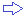with no precedence (i.e., multiplication doesn't automatically get computed before addition) .

2- Then the whole expression is evaluated from right to left.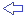``````>> square-root 2 + 2 + square-root 3 * 3 * square-root 1 + 4 * 5
== 3.272339214155429
``````### 3 concepts explanation:

This seems to work and I think that is how the interpreter does it, but is not a simple rule.

#### Concept 1: Left to rightIn Red, things are evaluated from left to right. There is no "order of precedence" as in other languages (i.e., multiplication doesn't automatically get computed before addition). However, you may enclose the functions in parentheses to force precedence.

``````>> 2 + 3 * 5
== 25                 ; not 17!
``````

Not only expressions, but the whole code of a program is evaluated from left to right.

#### Infix operators

"+", "-", "*", "/" are called infix operators. For a complete list of those see red-by-example.com. They are actually the functions `add` , `multiply`, `divide` and `subtract`, which need two arguments. So:

`3 + 2` is actually `add 3 2`

`5 * 8`is actually `multiply 5 8` ...

...and so on.

`2 + 3 * 5` is just a more readable form of `multiply add 2 3 5` . Red's interpreter does the conversion for you.

These special syntaxes created to simplify coding are common in programming languages and are called syntatic sugar.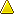There is an ongoing discussion on this, but currently my belief is that any expression with infix operators (except maybe "and" "or" "xor" and "is") can be "translated" to one, and only one, expression using the operation functions, and this is evaluated from left to right.

#### Concept 2: Evaluable groups.

When you have a chunk of code, there are groups of words that are evaluable, that is, can be reduced to basic datatypes. For example `[square-root 16 8 + 2 8 / 2 77]` is actually made of 4 evaluable groups: `square-root 16` ; `8 + 2` ; `8 / 2` and `77`. You can use `reduce` to "see" the values of evaluable groups:

``````>> a: [ square-root 16 8 + 2 8 / 2 77]
``````

####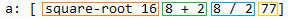``````>> reduce a
== [4.0 10 4 77]
``````

#### Concept 3: Functions pick their arguments from the evaluable groups

A function takes its arguments from the evaluable groups ahead of it, from left to right (think of infix operators as syntax sugar for their function counterparts). A function that needs 1 argument, take the next evaluable group; a function that needs 2 arguments, take the next 2 evaluable groups, and so on. Notice that a function may use an evaluable group that has another function in it. In this case, it holds its evaluation until the argument function is evaluated, and then use the result.

Again, no precedence rules, just left to right.

####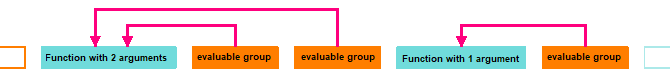A consequence of that is that an expression like this...

``````square-root 16 + square-root 16
``````

...is not 8, as many would expect, but 4.47213595499958, because what Red sees is:( or even: `square-root add 16 square-root 16`)

That is: One function that has one argument and one evaluable group (which happens to have a function in it).

To obtain that intuitive 8, one must use parentheses:

``````>> (square-root 16) + square-root 16
== 8.0
``````

Another example, mixing an infix operator and it's corresponding function:

``````>> reduce [add 8 + 2 * 3 8 / 2 divide 16 / 2 2 * 2]
== [34 2]
``````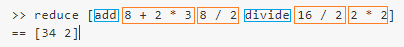### Other explanations:

#1

"Left-to-right and operators take precedence over functions and if an infix operator sees a function as its second operand, evaluates it"

#2

"In general, expressions are evaluated from left to right; however, within each expression evaluation occurs from right to left".

#3

"Each expression takes as many arguments as it should, each argument in turn may be another expression and Red will parse the expressions until they all have a full set of arguments".